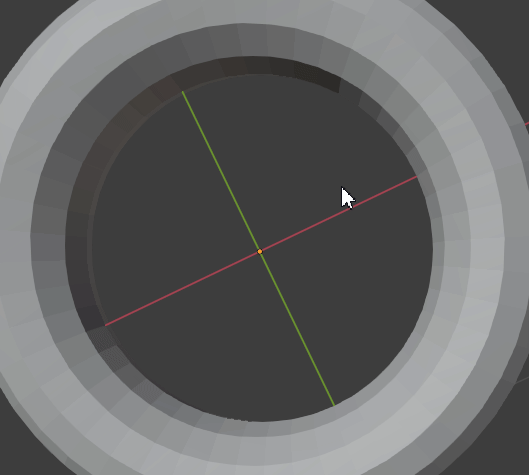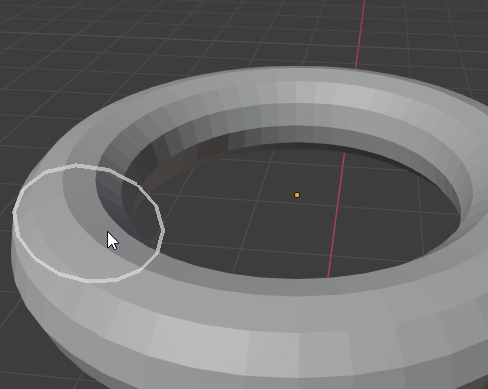# Need help with rotation + vector math

Hi, I am working on a modal that uses GPUshader to draw a circle.

` batch = batch_for_shader(shader, 'LINE_LOOP', {"pos": self.circle})`
I would like to not only rotate and align towards vector, but move the circle at the same time.

Here is the code that takes the circle and rotates it so it faces the Vector direction(target):

``````ob = bpy.context.active_object
matrix = ob.matrix_world

segments = 10
mul = (1.0 / (segments - 1)) * (pi * 2)

target = self.volume_snap # Vector

loc = Matrix.Translation(target)

DirectionVector = mathutils.Vector(target)

rot = DirectionVector.to_track_quat('Z')

self.circle = [ ( sin(i * mul)* radius, cos(i * mul) * radius , 0)
for i in range(segments)]

center =  sum((Vector(p) for p in self.circle), Vector()) / len(self.circle)

for i, p in enumerate(self.circle):
self.circle[i] = rot @ Vector(p) # how can i move the circle while it rotates?
``````I can rotate and move it individually but when adding them together, the only problem is it aligns towards the the world origin( Vector(0,0,0) )

And final result I am looking for:this was the old way, i used a real circle with vertices, but would like to convert to just gpushader draw handle

Maybe it’s the for loop that’s messing things up?

I wonder if you could actually get the current edge loop being raycasted and find center position. However this is only for good models with proper topology.

If your models have no topology, you would begin to step into the realm of predictive algorithms. Such as these semi-auto retopology tools that are able to convert a 2D raycast line into a 3D wrap and such.